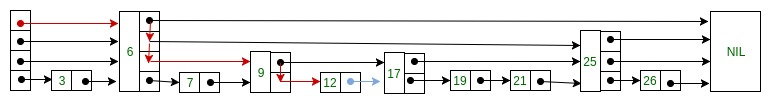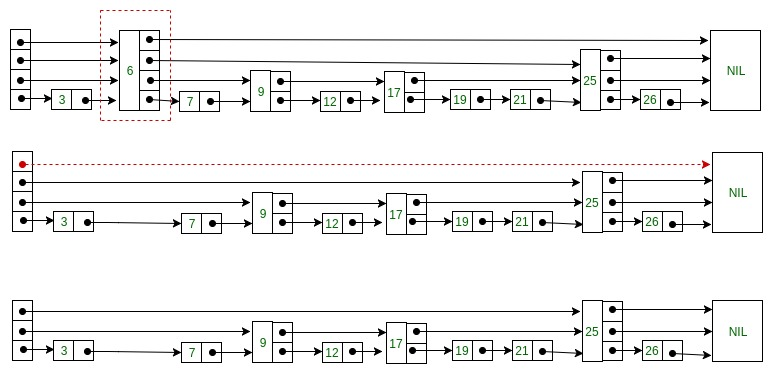Related Articles

# Skip List | Set 3 (Searching and Deletion)

• Difficulty Level : Hard
• Last Updated : 16 Feb, 2018

In previous article Skip List | Set 2 (Insertion) we discussed the structure of skip nodes and how to insert an element in the skip list. In this article we will discuss how to search and delete an element from skip list.

Searching an element in Skip list

Attention reader! Don’t stop learning now. Get hold of all the important DSA concepts with the DSA Self Paced Course at a student-friendly price and become industry ready.  To complete your preparation from learning a language to DS Algo and many more,  please refer Complete Interview Preparation Course.

In case you wish to attend live classes with experts, please refer DSA Live Classes for Working Professionals and Competitive Programming Live for Students.

Searching an element is very similar to approach for searching a spot for inserting an element in Skip list. The basic idea is if –

1. Key of next node is less than search key then we keep on moving forward on the same level.
2. Key of next node is greater than the key to be inserted then we store the pointer to current node i at update[i] and move one level down and continue our search.

At the lowest level (0), if the element next to the rightmost element (update) has key equal to the search key, then we have found key otherwise failure.
Following is the pseudo code for searching element –

```Search(list, searchKey)
-- loop invariant: x -> key  level downto 0 do
while x -> forward[i] -> key  forward[i]
x := x -> forward
if x -> key = searchKey then return x -> value
else return failure
```

Consider this example where we want to search for key 17-Deleting an element from the Skip list

Deletion of an element k is preceded by locating element in the Skip list using above mentioned search algorithm. Once the element is located, rearrangement of pointers is done to remove element form list just like we do in singly linked list. We start from lowest level and do rearrangement until element next to update[i] is not k.

After deletion of element there could be levels with no elements, so we will remove these levels as well by decrementing the level of Skip list. Following is the pseudo code for deletion –

```Delete(list, searchKey)
local update[0..MaxLevel+1]
for i := list -> level downto 0 do
while x -> forward[i] -> key  forward[i]
update[i] := x
x := x -> forward
if x -> key = searchKey then
for i := 0 to list -> level do
if update[i] -> forward[i] ≠ x then break
update[i] -> forward[i] := x -> forward[i]
free(x)
while list -> level > 0 and list -> header -> forward[list -> level] = NIL do
list -> level := list -> level – 1
```

Consider this example where we want to delete element 6 –Here at level 3, there is no element (arrow in red) after deleting element 6. So we will decrement level of skip list by 1.

Following is the code for searching and deleting element from Skip List –

## C++

 `// C++ code for searching and deleting element in skip list`` ` `#include ``using` `namespace` `std;`` ` `// Class to implement node``class` `Node``{``public``:``    ``int` `key;`` ` `    ``// Array to hold pointers to node of different level ``    ``Node **forward;``    ``Node(``int``, ``int``);``};`` ` `Node::Node(``int` `key, ``int` `level)``{``    ``this``->key = key;`` ` `    ``// Allocate memory to forward ``    ``forward = ``new` `Node*[level+1];`` ` `    ``// Fill forward array with 0(NULL)``    ``memset``(forward, 0, ``sizeof``(Node*)*(level+1));``};`` ` `// Class for Skip list``class` `SkipList``{``    ``// Maximum level for this skip list``    ``int` `MAXLVL;`` ` `    ``// P is the fraction of the nodes with level ``    ``// i pointers also having level i+1 pointers``    ``float` `P;`` ` `    ``// current level of skip list``    ``int` `level;`` ` `    ``// pointer to header node``    ``Node *header;``public``:``    ``SkipList(``int``, ``float``);``    ``int` `randomLevel();``    ``Node* createNode(``int``, ``int``);``    ``void` `insertElement(``int``);``    ``void` `deleteElement(``int``);``    ``void` `searchElement(``int``);``    ``void` `displayList();``};`` ` `SkipList::SkipList(``int` `MAXLVL, ``float` `P)``{``    ``this``->MAXLVL = MAXLVL;``    ``this``->P = P;``    ``level = 0;`` ` `    ``// create header node and initialize key to -1``    ``header = ``new` `Node(-1, MAXLVL);``};`` ` `// create random level for node``int` `SkipList::randomLevel()``{``    ``float` `r = (``float``)``rand``()/RAND_MAX;``    ``int` `lvl = 0;``    ``while``(r < P && lvl < MAXLVL)``    ``{``        ``lvl++;``        ``r = (``float``)``rand``()/RAND_MAX;``    ``}``    ``return` `lvl;``};`` ` `// create new node``Node* SkipList::createNode(``int` `key, ``int` `level)``{``    ``Node *n = ``new` `Node(key, level);``    ``return` `n;``};`` ` `// Insert given key in skip list``void` `SkipList::insertElement(``int` `key)``{``    ``Node *current = header;`` ` `    ``// create update array and initialize it``    ``Node *update[MAXLVL+1];``    ``memset``(update, 0, ``sizeof``(Node*)*(MAXLVL+1));`` ` `    ``/*    start from highest level of skip list``        ``move the current pointer forward while key ``        ``is greater than key of node next to current``        ``Otherwise inserted current in update and ``        ``move one level down and continue search``    ``*/``    ``for``(``int` `i = level; i >= 0; i--)``    ``{``        ``while``(current->forward[i] != NULL &&``              ``current->forward[i]->key < key)``            ``current = current->forward[i];``        ``update[i] = current;``    ``}`` ` `    ``/* reached level 0 and forward pointer to ``       ``right, which is desired position to ``       ``insert key. ``    ``*/``    ``current = current->forward;`` ` `    ``/* if current is NULL that means we have reached``       ``to end of the level or current's key is not equal``       ``to key to insert that means we have to insert``       ``node between update and current node */``    ``if` `(current == NULL || current->key != key)``    ``{``        ``// Generate a random level for node``        ``int` `rlevel = randomLevel();`` ` `        ``/* If random level is greater than list's current``           ``level (node with highest level inserted in ``           ``list so far), initialize update value with pointer``           ``to header for further use */``        ``if``(rlevel > level)``        ``{``            ``for``(``int` `i=level+1;iforward[i] = update[i]->forward[i];``            ``update[i]->forward[i] = n;``        ``}``        ``cout<<``"Successfully Inserted key "``<= 0; i--)``    ``{``        ``while``(current->forward[i] != NULL  &&``              ``current->forward[i]->key < key)``            ``current = current->forward[i];``        ``update[i] = current;``    ``}`` ` `    ``/* reached level 0 and forward pointer to ``       ``right, which is possibly our desired node.*/``    ``current = current->forward;`` ` `    ``// If current node is target node``    ``if``(current != NULL and current->key == key)``    ``{``        ``/* start from lowest level and rearrange``           ``pointers just like we do in singly linked list``           ``to remove target node */``        ``for``(``int` `i=0;i<=level;i++)``        ``{``            ``/* If at level i, next node is not target ``               ``node, break the loop, no need to move ``              ``further level */``            ``if``(update[i]->forward[i] != current)``                ``break``;`` ` `            ``update[i]->forward[i] = current->forward[i];``        ``}`` ` `        ``// Remove levels having no elements ``        ``while``(level>0 &&``              ``header->forward[level] == 0)``            ``level--;``         ``cout<<``"Successfully deleted key "``<= 0; i--)``    ``{``        ``while``(current->forward[i] &&``               ``current->forward[i]->key < key)``            ``current = current->forward[i];`` ` `    ``}`` ` `    ``/* reached level 0 and advance pointer to ``       ``right, which is possibly our desired node*/``    ``current = current->forward;`` ` `    ``// If current node have key equal to``    ``// search key, we have found our target node``    ``if``(current and current->key == key) ``        ``cout<<``"Found key: "``<forward[i];``        ``cout<<``"Level "``<key<<``" "``;``            ``node = node->forward[i];``        ``}``        ``cout<<``"\n"``;``    ``}``};`` ` `// Driver to test above code``int` `main()``{``    ``// Seed random number generator``    ``srand``((unsigned)``time``(0));`` ` `    ``// create SkipList object with MAXLVL and P ``    ``SkipList lst(3, 0.5);`` ` `    ``lst.insertElement(3);``    ``lst.insertElement(6);``    ``lst.insertElement(7);``    ``lst.insertElement(9);``    ``lst.insertElement(12);``    ``lst.insertElement(19);``    ``lst.insertElement(17);``    ``lst.insertElement(26);``    ``lst.insertElement(21);``    ``lst.insertElement(25);``    ``lst.displayList();`` ` `    ``//Search for node 19``    ``lst.searchElement(19);`` ` `    ``//Delete node 19``    ``lst.deleteElement(19);``    ``lst.displayList();``}`

## Python

 `# Python3 code for searching and deleting element in skip list`` ` `import` `random`` ` `class` `Node(``object``):``    ``'''``    ``Class to implement node``    ``'''``    ``def` `__init__(``self``, key, level):``        ``self``.key ``=` `key`` ` `        ``# list to hold references to node of different level ``        ``self``.forward ``=` `[``None``]``*``(level``+``1``)`` ` `class` `SkipList(``object``):``    ``'''``    ``Class for Skip list``    ``'''``    ``def` `__init__(``self``, max_lvl, P):``        ``# Maximum level for this skip list``        ``self``.MAXLVL ``=` `max_lvl`` ` `        ``# P is the fraction of the nodes with level ``        ``# i references also having level i+1 references``        ``self``.P ``=` `P`` ` `        ``# create header node and initialize key to -1``        ``self``.header ``=` `self``.createNode(``self``.MAXLVL, ``-``1``)`` ` `        ``# current level of skip list``        ``self``.level ``=` `0``     ` `    ``# create  new node``    ``def` `createNode(``self``, lvl, key):``        ``n ``=` `Node(key, lvl)``        ``return` `n``     ` `    ``# create random level for node``    ``def` `randomLevel(``self``):``        ``lvl ``=` `0``        ``while` `random.random()<``self``.P ``and` `\``              ``lvl<``self``.MAXLVL:lvl ``+``=` `1``        ``return` `lvl`` ` `    ``# insert given key in skip list``    ``def` `insertElement(``self``, key):``        ``# create update array and initialize it``        ``update ``=` `[``None``]``*``(``self``.MAXLVL``+``1``)``        ``current ``=` `self``.header`` ` `        ``'''``        ``start from highest level of skip list``        ``move the current reference forward while key ``        ``is greater than key of node next to current``        ``Otherwise inserted current in update and ``        ``move one level down and continue search``        ``'''``        ``for` `i ``in` `range``(``self``.level, ``-``1``, ``-``1``):``            ``while` `current.forward[i] ``and` `\``                  ``current.forward[i].key < key:``                ``current ``=` `current.forward[i]``            ``update[i] ``=` `current`` ` `        ``''' ``        ``reached level 0 and forward reference to ``        ``right, which is desired position to ``        ``insert key.``        ``'''` `        ``current ``=` `current.forward[``0``]`` ` `        ``'''``        ``if current is NULL that means we have reached``           ``to end of the level or current's key is not equal``           ``to key to insert that means we have to insert``           ``node between update and current node``       ``'''``        ``if` `current ``=``=` `None` `or` `current.key !``=` `key:``            ``# Generate a random level for node``            ``rlevel ``=` `self``.randomLevel()`` ` `            ``'''``            ``If random level is greater than list's current``            ``level (node with highest level inserted in ``            ``list so far), initialize update value with reference``            ``to header for further use``            ``'''``            ``if` `rlevel > ``self``.level:``                ``for` `i ``in` `range``(``self``.level``+``1``, rlevel``+``1``):``                    ``update[i] ``=` `self``.header``                ``self``.level ``=` `rlevel`` ` `            ``# create new node with random level generated``            ``n ``=` `self``.createNode(rlevel, key)`` ` `            ``# insert node by rearranging references ``            ``for` `i ``in` `range``(rlevel``+``1``):``                ``n.forward[i] ``=` `update[i].forward[i]``                ``update[i].forward[i] ``=` `n`` ` `            ``print``(``"Successfully inserted key {}"``.``format``(key))`` ` `    ``def` `deleteElement(``self``, search_key):`` ` `        ``# create update array and initialize it``        ``update ``=` `[``None``]``*``(``self``.MAXLVL``+``1``)``        ``current ``=` `self``.header`` ` `        ``'''``        ``start from highest level of skip list``        ``move the current reference forward while key ``        ``is greater than key of node next to current``        ``Otherwise inserted current in update and ``        ``move one level down and continue search``        ``'''``        ``for` `i ``in` `range``(``self``.level, ``-``1``, ``-``1``):``            ``while``(current.forward[i] ``and` `\``                  ``current.forward[i].key < search_key):``                ``current ``=` `current.forward[i]``            ``update[i] ``=` `current`` ` `        ``''' ``        ``reached level 0 and advance reference to ``        ``right, which is prssibly our desired node``        ``'''` `        ``current ``=` `current.forward[``0``]`` ` `        ``# If current node is target node``        ``if` `current !``=` `None` `and` `current.key ``=``=` `search_key:`` ` `            ``'''``            ``start from lowest level and rearrange references ``            ``just like we do in singly linked list``            ``to remove target node``            ``'''``            ``for` `i ``in` `range``(``self``.level``+``1``):`` ` `                ``'''``                ``If at level i, next node is not target ``                ``node, break the loop, no need to move ``                ``further level``                ``'''``                ``if` `update[i].forward[i] !``=` `current:``                    ``break``                ``update[i].forward[i] ``=` `current.forward[i]`` ` `            ``# Remove levels having no elements``            ``while``(``self``.level>``0` `and``\``                  ``self``.header.forward[``self``.level] ``=``=` `None``):``                ``self``.level ``-``=` `1``            ``print``(``"Successfully deleted {}"``.``format``(search_key))`` ` `    ``def` `searchElement(``self``, key): ``        ``current ``=` `self``.header`` ` `        ``'''``        ``start from highest level of skip list``        ``move the current reference forward while key ``        ``is greater than key of node next to current``        ``Otherwise inserted current in update and ``        ``move one level down and continue search``        ``'''``        ``for` `i ``in` `range``(``self``.level, ``-``1``, ``-``1``):``            ``while``(current.forward[i] ``and``\``                  ``current.forward[i].key < key):``                ``current ``=` `current.forward[i]`` ` `        ``# reached level 0 and advance reference to ``        ``# right, which is prssibly our desired node``        ``current ``=` `current.forward[``0``]`` ` `        ``# If current node have key equal to``        ``# search key, we have found our target node``        ``if` `current ``and` `current.key ``=``=` `key:``            ``print``(``"Found key "``, key)`` ` ` ` `    ``# Display skip list level wise``    ``def` `displayList(``self``):``        ``print``(``"\n*****Skip List******"``)``        ``head ``=` `self``.header``        ``for` `lvl ``in` `range``(``self``.level``+``1``):``            ``print``(``"Level {}: "``.``format``(lvl), end``=``" "``)``            ``node ``=` `head.forward[lvl]``            ``while``(node !``=` `None``):``                ``print``(node.key, end``=``" "``)``                ``node ``=` `node.forward[lvl]``            ``print``("")`` ` `# Driver to test above code``def` `main():``    ``lst ``=` `SkipList(``3``, ``0.5``)``    ``lst.insertElement(``3``)``    ``lst.insertElement(``6``)``    ``lst.insertElement(``7``)``    ``lst.insertElement(``9``)``    ``lst.insertElement(``12``)``    ``lst.insertElement(``19``)``    ``lst.insertElement(``17``)``    ``lst.insertElement(``26``)``    ``lst.insertElement(``21``)``    ``lst.insertElement(``25``)``    ``lst.displayList()`` ` `    ``# Search for node 19``    ``lst.searchElement(``19``)`` ` `    ``# Delete node 19``    ``lst.deleteElement(``19``)``    ``lst.displayList()`` ` `main()`

Output:

```Successfully Inserted key 3
Successfully Inserted key 6
Successfully Inserted key 7
Successfully Inserted key 9
Successfully Inserted key 12
Successfully Inserted key 19
Successfully Inserted key 17
Successfully Inserted key 26
Successfully Inserted key 21
Successfully Inserted key 25

*****Skip List*****
Level 0: 3 6 7 9 12 17 19 21 25 26
Level 1: 3 17 19 21 26
Level 2: 17 19 21
Found key: 19
Successfully deleted key 19

*****Skip List*****
Level 0: 3 6 7 9 12 17 21 25 26
Level 1: 3 17 21 26
Level 2: 17 21
```

Time complexity of both searching and deletion is same –
Time complexity (Average):Time complexity (Worst):This article is contributed by Atul Kumar. If you like GeeksforGeeks and would like to contribute, you can also write an article using contribute.geeksforgeeks.org or mail your article to contribute@geeksforgeeks.org. See your article appearing on the GeeksforGeeks main page and help other Geeks.